﻿ New Methods for Comparing the Forecasts Accuracy

New Methods for Comparing the Forecasts Accuracy

Bratu (Simionescu) MihaelaOPEN ACCESSPEER-REVIEWED

New Methods for Comparing the Forecasts Accuracy

Bratu (Simionescu) MihaelaDepartment of Statistics and Econometrics, Faculty of Cybernetics, Statistics and Economic Informatics, Bucharest, Romania

Abstract

The main purpose of this research is to show the diversity of statistical methods that could be used to assess and compare forecasts accuracy. Some of the statistical approaches were not used before in literature to evaluate the forecasts accuracy. The different methods applied to compare the accuracy of the USA inflation forecasts on the horizon 1976-2012 started from the predictions provided by Survey of Professional Forecasters (SPF), Congressional Budget Office (CBO), Blue Chips (BC), and Administration, determining different results. According to U1 Theil's statistic, non-parametric tests and a new indicator proposed by us (RRSSE- ratio of radicals of sum of squared errors), the best forecasts were provided by Administration and the less accurate by SPF. The Spearman's and Kendall's coefficients of correlation and the ranks method gavea hierarchy of institutions performance regarding the accuracy that starts with BC and finished with SPF. The logistic regression computed by the author and the relative distance to the maximal performance method considered CBO as the best institution. Some methods of improving the forecasts accuracy were applied, getting more accurate predictions for the combined forecasts of BC and CBO using optimal scheme of combination. The smoothed predicted values based on Hodrick-Prescott filter outperformed all the initial predictions and the combined ones.

• Mihaela, Bratu (Simionescu). "New Methods for Comparing the Forecasts Accuracy." American Journal of Applied Mathematics and Statistics 1.1 (2013): 1-5.
• Mihaela, B. (. (2013). New Methods for Comparing the Forecasts Accuracy. American Journal of Applied Mathematics and Statistics, 1(1), 1-5.
• Mihaela, Bratu (Simionescu). "New Methods for Comparing the Forecasts Accuracy." American Journal of Applied Mathematics and Statistics 1, no. 1 (2013): 1-5.

 Import into BibTeX Import into EndNote Import into RefMan Import into RefWorks

1. Introduction

An auxiliary, but essential component of the forecasting process is the assessment of the accuracy, which reflects how closer the forecasted values of a variable are to its registered values. In USA there are more institutions that provide predictions for macroeconomic indicators. The main question is which of these institutions predicted the best an economic phenomenon. To answer this question we can use many methods. Some of the usual statistical methods were not applied in the forecasting context. It is important to analyze if more methods gave the same results. On the other hand, it is also essential to find out some empirical strategies to improve the forecasts accuracy.

In this study the accuracy is assessed in ex-post variant, resulting a mirror of the historical accuracy of institutions forecasts. Consequently, this analyze will be the best guide to choose the forecasts of a certain institution in the close future.

2. New Statistical Methods Used in Making Comparisons between Predictions

Some researchers were interested in evaluating the accuracy of macroeconomic forecasts made by some international institutions. However, they omitted to take into account the Administration anticipations.

Edge, Kiley and Laforte assessed the accuracy of predictions made by Federal Reserve staff and for those made starting from a DSGE model and a time-series model .

Abreu were interested in assessing the accuracy of predictions made by the Organization for Economic Co-operation and Development (OECD), International Monetary Fund ( IMF), European Commission (EC) and two private institutions (Consensus Economics and The Economist) . The directional accuracy and the ability of anticipating an economic crisis were deeply studied. The probability of USA recession was computed by Österholm (2012) starting from a BVAR model .

In general, the researchers used the classical measures of accuracy, like mean error, mean absolute errors, root mean squared error, U Theil’s coefficient. Percentages errors, U statistics or mean absolute scaled errors are used to make comparisons in terms of accuracy.

One problem that we try to solve in this study is to bring an objective classification of forecasts. In practice, some of the accuracy measures recommend as better a certain forecast, while others show that other forecast is more accurate. In order to solve this uncertain situation we applied the multi-criteria methods that take into account at the same time the values of all accuracy indicators.

The ranks method and the method of relative distance with respect to the maximal performance are applied to order the forecasts according to accuracy criterion.

For the forecasted variable X, the error is calculated as the difference between the real value and the predicted one. It is denoted by “e”. Some of the measures of predictions accuracy are presented beWe selected 5 accuracy measures whose influence is taken into account at the same time.

If n is the length of the forecast horizon, then we computed:

1. The Mean error (ME)(1)

2. The Mean absolute error (MAE)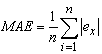(2)

3. The Root Mean Squared Error (RMSE)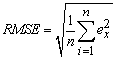(3)

4. The U1 Theil’s statistic(4)

r- the real values; f- the forecasted values

A higher accuracy is equivalent with a value closer to zero for U1 statistic.

5. U2 Theil’s statistic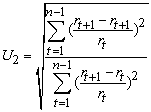(5)

A value less than 1 for U2 confirms the superiority of the compared forecast, while a value greater than 1 shows a higher acccuracy for the benchmark forecast.

For comparisons with the naive forecasts a new indicator is introduced by us: ratio of radicals of sum of squared errors (RRSSE).In order to compare two predictions even for different variables, the values of this indicator are compared, a value closer to zero showing a better accuracy.

Ranks method has several steps:

1. Each accuracy measure receives a rank according to its value. (the value that indicates the a better degree accuracy has the rank 1);

The statistical units are represented by the number of institutions that provided forecasts. In our case study this number is 3. The rank corresponding to each institution is:and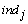-accuracy indicator j.

The ranks are sum up and institution with the lowest score receives the rank one:(6)

2. The institution with the lowest score is the best one and it gets the final rank 1.

The method of relative distance related to the maximal performance supposes that for each accuracy measure the distance of each institution compared to the one with the best performance is calculated as:(7)

The relative distance is calculated as a ratio, where the denominator is the lowest value of the accuracy indicator for all institutions.

The geometric mean for the relative distances is calculated: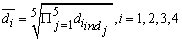(8)

The final ranks are assigned taking into account the values of average relative distances. The institution with the lowest average relative distance receives the rank 1. The location of each institution compared to the one with the best performance is a ratio: the average relative distance over the lowest average relative distance.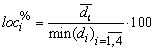(9)

Wilcoxon Signed Ranks test and Kruskall-Wallis test are nonparametric tests used when the series repartition is not known or non-normal. These tests are applied to check the differences between populations. In this case, the differences between the real values and the forecasted ones are checked using the two non-parametric tests. The null hypothesis refers to the lack of differences, while the alternative one shows that there are significant differences between the forecasts and the registered values. A p-value that is lower than 0.05 implies the rejection of null hypothesis. For small samples the chi-square approximation gives better results in most cases than Kruskal-Wallis test, according to Conover .

Comparisons between forecasts can be done using binary logistic regression when the dependent variable is a qualitative one. For this type of regression some assumptions are not considered (errors non-correlation, normality or homoscedasticity).Odd-ratios (OR) are computed to see how much the occurrence chances of an alternative of the dependent variable modify when the independent variable change with one unit. The coefficient of the exogenous variable from the regression model is denoted by b1.

If OR is higher than 1, an increase by one unit in the level of the exogenous variable implies a growth by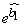in the level of the dependent variable.

The absolute errors for each year in the forecasting horizon are computed. We test is each error differs significantly from a threshold fixed at 0.5%. We choose a threshold of 1%. The dependent variable has two alternatives:

error_significance=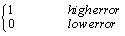For each forecasted value of the variable, the significance of the error is computed.

The Spearman’s and Kendall’s coefficients of correlation might be computed to see the associations between the real values and the predicted ones.

3. Inflation Forecasts Comparisons for USA

The forecasting horizon for USA inflation rate is 1978-2012 and the predictions are provided by Survey of Professional Forecasters (SPF), Congressional Budget Official (CBO), Blue Chips (BC) and Administration.

It is not recommend the use of a single measure of accuracy. In our study 5 accuracy indicators were selected.

Table 1. The accuracy of inflation forecasts provided by SPF, CBO, BC and Administration (1978-2012)

According to U1 statistic, the best forecasts are provided by Administration, being followed by BCanticipations, CBO ones and finally the SPF forecasts. However, CBO predictions have the lowest mean error. Administration registered the lowest mean absolute error for their predictions. Only the SPF anticipations are better than the naïve forecasts. The indicator RRSSE introduced by us in literature gave the same results as U1.

The multi-criteria ranking solves the problem of contradictory measures of accuracy by considering their influence at the same time.

Table 2. The ranks method for the comparison of USA inflation forecasts accuracy (1976-2012)

The ranks method recommends BC forecasts as the best and the SPF as the less accurate. CBO and Administration predictions have the same degree of accuracy.

According to the second method of multi-criteria ranking, the best forecasts on the horizon 1976-2012 were provided by CBO. The hierarchy of institutions is continued by: SPF, BC and Administration. So, there are differences regarding the hierarchy provided by the two methods. In general, the method of relative distance according to the best institution gives better results. However, CBO gave the best performance according to both methods.

Table 3. The method of relative distance related to the maximal performance for the comparison of USA inflation forecasts accuracy (1976-2012)

The dependencies between the effective values and the forecasted ones are analyzed using non-parametric tests like Wilcoxon Sum Ranks and Kruskall-Wallis.

After the application of non-parametric tests we made the following conclusions with a probability of 95%:

The differences between CBO predictions and the registered values are not significant;

The differences between SPF predictions and the real values are not significant, but the Significance indicator is lower; this shows that CBO predictions are better than the SPF ones;

The differences are not significant between BC forecasts and the real values, but the p-valueis lower than that of the other two predictions; this implies that SPF and CBO expectations are better than BC ones;

There are significant differences between Administration forecasts and the effective values on inflation. The results of the tests applied in SAS are presented in Appendix 1.

So, the hierarchy given by the application of non-parametric tests is: Administration, CBO and Blue Chips. For all the SPF predictions the errors are significant and larger than de threshold of 1%.

The odds of having a low error for CBO forecasts grow with 36.6%, while the chances for Blue Chips increase with 25.3%. For Administration forecasts only few errors are not significant. So, the hierarchy provided by the analysis of binary logistic regression is: CBO, Blue Chips, Administration and SPF. The results of this procedure are displayed in Appendix 2.

The Spearman’s and Kendall’s coefficients of correlation are computed in Appendix 3. The strong correlation is between BC forecasts and real value, being followed by the one between Administration and the effective values and CBO and real values. The correlation between SPF expectation and the real values is not significant.

4. Strategies of Improving the Forecasts Accuracy

Bratu (2012) specified some strategies of improving the forecasts accuracy (application of filters and exponential smoothing techniques, combined forecasts, regressions models, historical errors method).

The most use approaches for combined forecasts are: optimal combination (OPT), inverse MSE weighting scheme (INV) and equal-weights-scheme (EW).

Bates and Granger) used two forecasts f1;t and f2;t, of the same variable Xt, derived h periods ago. For unbiased forecasts, the error is computed as:. The errors follow a normal repartition of parameters 0 and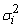. If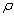is the errors coefficient of correlation the covariance is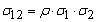. The linear combination of the two predictions is: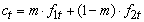. The error of the combined forecast is: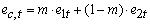.The combined forecast mean is zero and the variance is: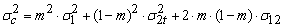The optimal value for m is determined by minimizing the error variance (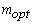) :(10)

The inverse weight (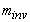) is computed as: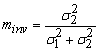(11)

For equally weighted combined predictions (EW) the same weights are given to all models.

The U1 Theil’s coefficient is computed for the combined forecasts based on the three schemes.

Table 4. The accuracy of USA inflation combined forecasts on the horizon 1976-2012

The combined forecasts of CBO and BC using OPT scheme improved the accuracy of all predictions. This type of combination gave better results than all the initial forecasts, excepting Administration ones. All the other combined forecasts excepting those where SPF anticipations are implied are better than SPF and CBO ones.

The application of filters to the initial forecasts and also the exponential smoothing techniques as Holts Winters are utilized for improving the forecasts accuracy .

The Hodrick–Prescott (HP) filter is used to extract the trend of the data series. Razzak explained that the Hodrick-Prescott filteris a true `filter' at the end of the sample and a `smoother' one over the sample . The output gap from the true filter determines more accurate out-of-sample predictions of inflation. Christiano and Fitzgerald showed that Band-Pass filter is used to determine the component of the time series that is situated within a specific band of frequencies . Christiano-Fitzegerald filter (CF filter) converges on long run to an optimal filter. It has a steep frequency response function at the band boundaries.

Holt-Winters (HW) Simple exponential smoothing technique is recommended for data set with linear trend and no seasonal variations. The filters and the HW technique are utilized to smooth the predictions provided by the four institutions. Then, the accuracy of the new forecasts is evaluated.

Table 5. The U1 values of USA inflation forecasts on the horizon 1976-2012

The Hodrick-Prescott technique and the Holt-Winters model improved the accuracy of BC and CBO forecasts. The great improvement was generated by Hodrick-Prescott filter for both types of predictions. For CBO anticipations, the accuracy is even better than that of the predictions provided by the other institutions or by the combined forecasts.

5. Conclusions

This research enriches the literature regarding the assessment and the improvement of forecasts accuracy.

According to U1 statistic and the new introduced RRSSE indicator and according to ranks method and to Spearman’s coefficient of correlation the hierarchy of institutions that forecasted between the two-year inflation in 1982-2011 is: CBO, Administration and Blue Chips. The relative distance method with respect to the better institution, the logistic regression, the non-parametric tests provided the following ranking: Administration, CBO and Blue Chips. The highest improvement in accuracy was brought by the combined forecasts of Blue Chips and Administration using inverse MSE scheme. The smoothed predicted values based on Holt-Winters technique, Hodrick-Prescott, Baxter King and Christiano-Fitzegerald filters din not improve the forecasts accuracy.

The novelty of this research consists in the application of some statistical approaches to compare the predictions accuracy, these methods never being mentioned in literature in this context. The results of the new approach are better than those provided by the U Theil’s statistic, because more aspects of accuracy problem are taken into account.

References

  Abreu I., “International organizations’ vs. private analysts’ forecasts: an Evaluation”, Banco de Portugal Papers, 29-34, 2011.In article  Bates, J., and C. W. J. Granger, “The Combination of Forecasts”, Operations Research Quarterly, 20(4): 451-468, Jul. 1969.In article  Bratu, M. (2012). Strategies to Improve the Accuracy of Macroeconomic Forecasts in USA, LAP LAMBERT Academic Publishing, 2012, 6-28.In article  Bratu (Simionescu) M.,“Filters or Holt Winters technique to improve the forecasts for USA inflation rate ?”, ActaUniversitatisDanubius. Œconomica, 9(1): 23-45, 2013.In article  Christiano, L. J. and Fitzgerald, T.J., “The Band Pass Filter”,International Economic Review, 44(2): 435-465, 2003.In article  Conovor W.J., Practical nonparametric statistics, Wiley series in probability and statistics, New York: Wiley, 1999, 56-78.In article  Edge R.M., Kiley M.T. and Laforte J.-P., “A comparison of forecast performance between Federal Reserve Staff Forecasts simple reduced-form models and a DSGE model”,Finance and Economics Discussion Series, 85-89, 2009.In article  Hodrick R. and Prescott, E.C., Postwar U.S. Business Cycles: An empirical investigation,Journal of Money, Credit and Banking, 1(16): 84-90, 2003.In article  Österholm, P., “The limited usefulness of macroeconomic Bayesian VARs when estimating the probability of a US recession”, Journal of Macroeconomics, Elsevier, vol. 34(1): 76-86, 2012.In article  RazzakW.,The Hodrick-Prescott technique: A smoother versus a filter: An application to New Zealand GDP, Economics Letters, 57(2), 163-168, 1997.In article  http://www.cbo.gov/sites/default/files/cbofiles/ftpdocs/115xx/doc11553/forecastingaccuracy.pdf.In article